# Velocity vs time graph How fast and where

• Slides: 25
Download presentation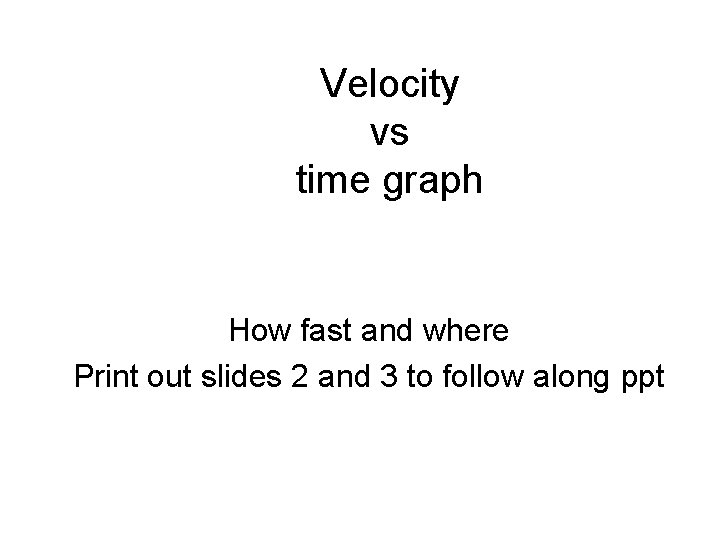Velocity vs time graph How fast and where Print out slides 2 and 3 to follow along ppt+15 m/s velocity +10 m/s +5 m/s 0 m/s -5 m/s -10 m/s 0 s 1 s 2 s time 3 s 4 s 5 s 6Questions 1. How fast is the object moving at 1 s? How fast is it moving at 1. 5 s ? 2. How fast is the object moving at 2. 5 s ? How fast is it moving at 3. 5 s ? 3. How fast is the object moving at 4. 5 s? How fast is it moving at 5. 0 s? 5. 5 s? 4. When did the object travel at constant velocity? What was the velocity? 6. When did the object speed up? How much did it speed up in the time interval? 7. When did the object slow down? When did it stop? 8. When did it have Positive velocity? When did it have negative velocity? When did it turn around?1. How fast is the object moving at 1 s? How fast is it moving at 1. 5 s ? +15 m/s velocity +10 m/s +5 m/s 0 m/s -5 m/s -10 m/s 0 s 1 s 2 s time 3 s 4 s 5 s 61. How fast is the object moving at 1 s? How fast is it moving at 1. 5 s ? +15 m/s At 1 s , it is moving at +5 m/s At 1. 5 s it is traveling at +5 m/s velocity +10 m/s +5 m/s 0 m/s -5 m/s -10 m/s 0 s 1 s 2 s time 3 s 4 s 5 s 6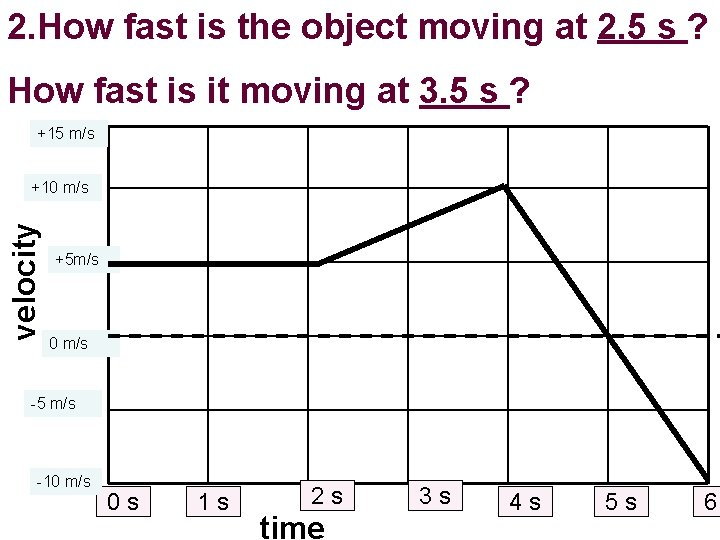2. How fast is the object moving at 2. 5 s ? How fast is it moving at 3. 5 s ? +15 m/s velocity +10 m/s +5 m/s 0 m/s -5 m/s -10 m/s 0 s 1 s 2 s time 3 s 4 s 5 s 6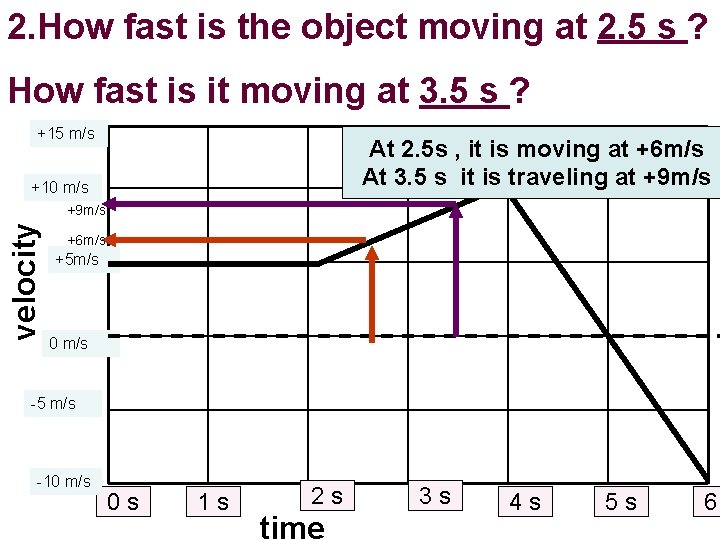2. How fast is the object moving at 2. 5 s ? How fast is it moving at 3. 5 s ? +15 m/s At 2. 5 s , it is moving at +6 m/s At 3. 5 s it is traveling at +9 m/s +10 m/s velocity +9 m/s +6 m/s +5 m/s 0 m/s -5 m/s -10 m/s 0 s 1 s 2 s time 3 s 4 s 5 s 6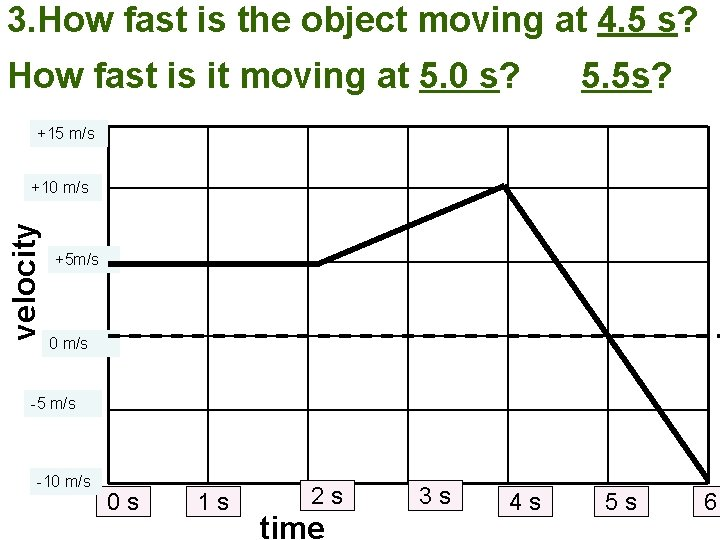3. How fast is the object moving at 4. 5 s? How fast is it moving at 5. 0 s? 5. 5 s? +15 m/s velocity +10 m/s +5 m/s 0 m/s -5 m/s -10 m/s 0 s 1 s 2 s time 3 s 4 s 5 s 63. How fast is the object moving at 4. 5 s? How fast is it moving at 5. 0 s? +15 m/s velocity +10 m/s 5. 5 s? At 4. 5 s , it is moving at +5 m/s At 5. 0 s it is traveling at 0 m/s At 5. 5 s it is traveling at -5 m/s +5 m/s 0 m/s -5 m/s -10 m/s 0 s 1 s 2 s time 3 s 4 s 5 s 64. When did the object travel at constant velocity? What was the velocity? +15 m/s velocity +10 m/s +5 m/s 0 m/s -5 m/s -10 m/s 0 s 1 s 2 s time 3 s 4 s 5 s 6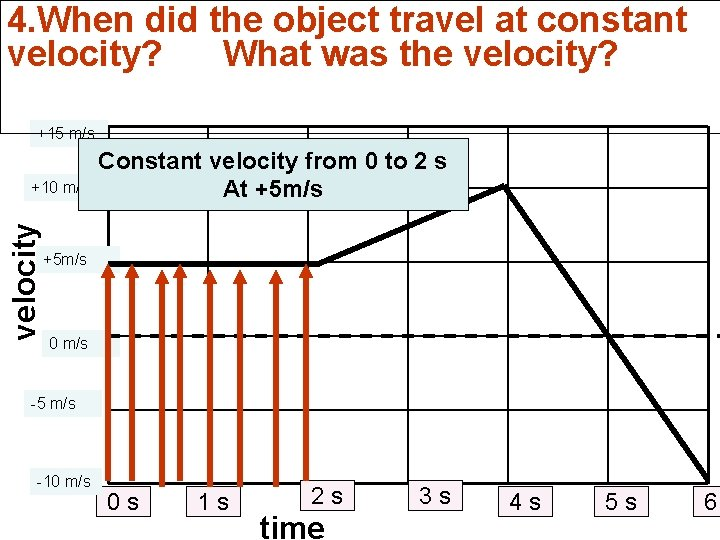4. When did the object travel at constant velocity? What was the velocity? +15 m/s velocity +10 m/s Constant velocity from 0 to 2 s At +5 m/s 0 m/s -5 m/s -10 m/s 0 s 1 s 2 s time 3 s 4 s 5 s 6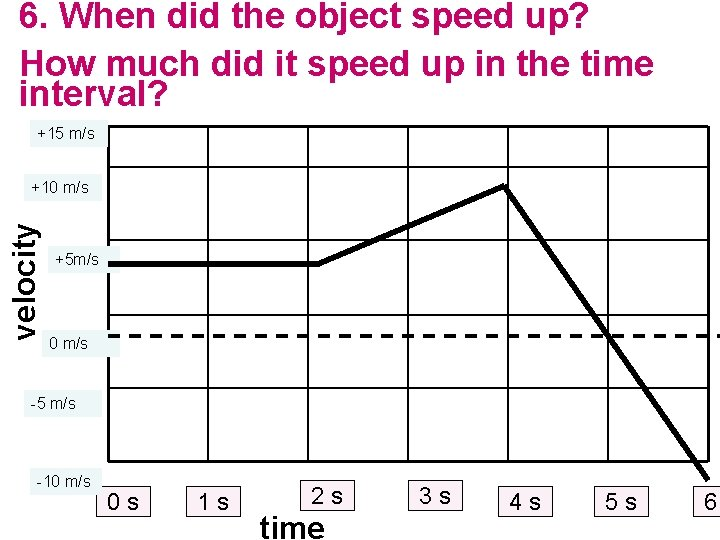6. When did the object speed up? How much did it speed up in the time interval? +15 m/s velocity +10 m/s +5 m/s 0 m/s -5 m/s -10 m/s 0 s 1 s 2 s time 3 s 4 s 5 s 6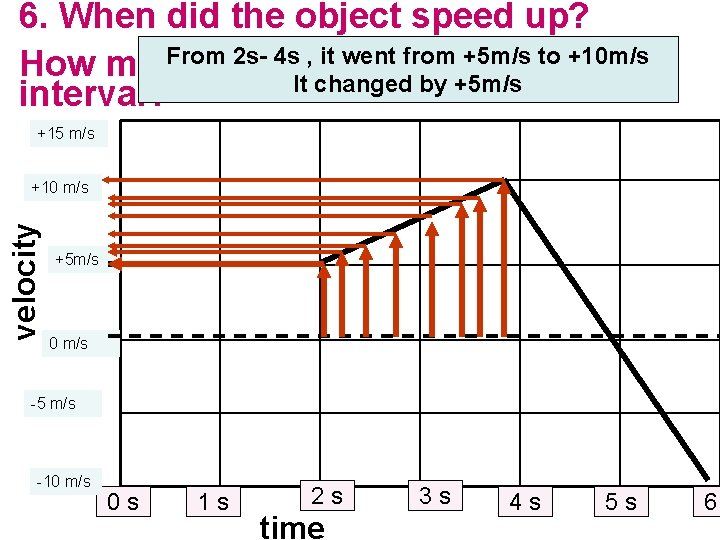6. When did the object speed up? From 2 s- 4 s , it went from +5 m/s to +10 m/s How much did it. Itspeed up in the time changed by +5 m/s interval? +15 m/s velocity +10 m/s +5 m/s 0 m/s -5 m/s -10 m/s 0 s 1 s 2 s time 3 s 4 s 5 s 6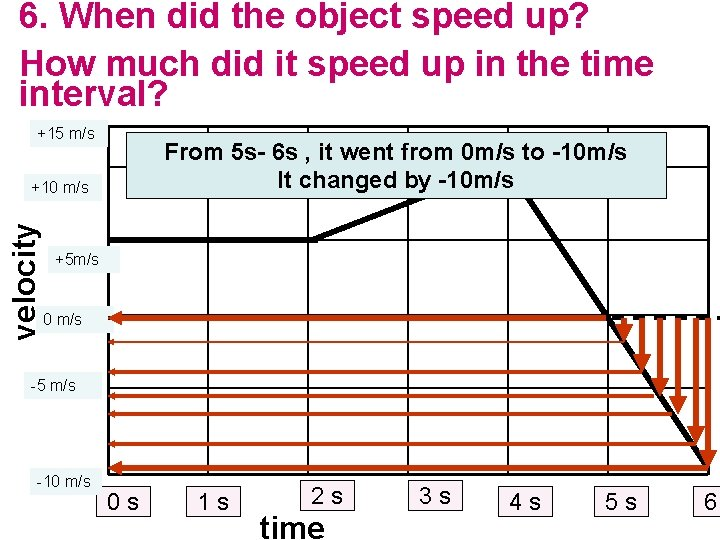6. When did the object speed up? How much did it speed up in the time interval? +15 m/s From 5 s- 6 s , it went from 0 m/s to -10 m/s It changed by -10 m/s velocity +10 m/s +5 m/s 0 m/s -5 m/s -10 m/s 0 s 1 s 2 s time 3 s 4 s 5 s 6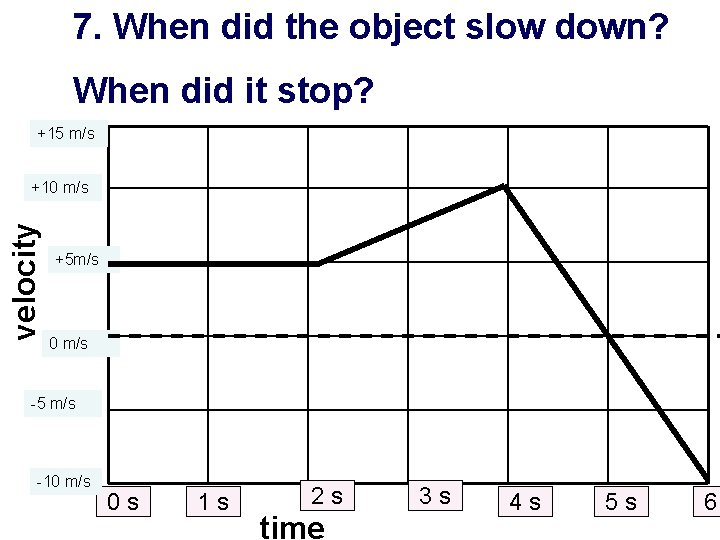7. When did the object slow down? When did it stop? +15 m/s velocity +10 m/s +5 m/s 0 m/s -5 m/s -10 m/s 0 s 1 s 2 s time 3 s 4 s 5 s 6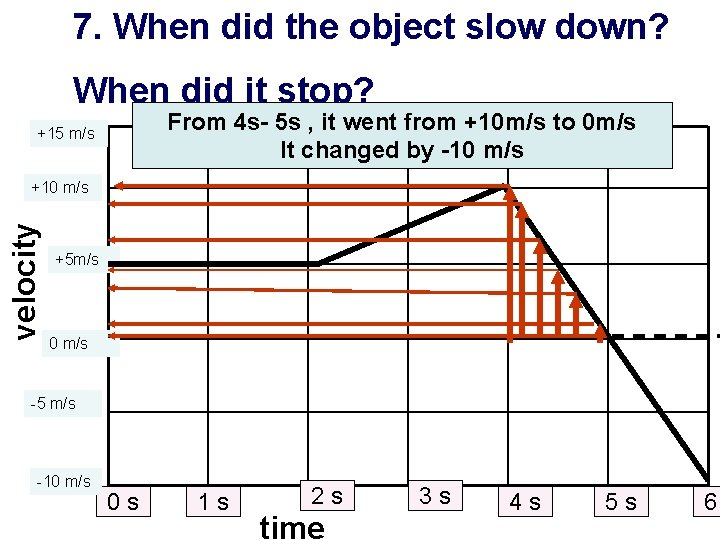7. When did the object slow down? When did it stop? From 4 s- 5 s , it went from +10 m/s to 0 m/s It changed by -10 m/s +15 m/s velocity +10 m/s +5 m/s 0 m/s -5 m/s -10 m/s 0 s 1 s 2 s time 3 s 4 s 5 s 6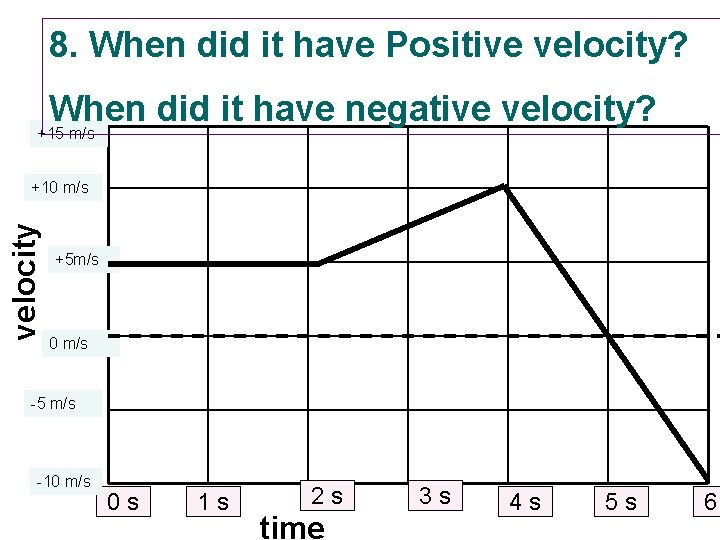8. When did it have Positive velocity? When did it have negative velocity? +15 m/s velocity +10 m/s +5 m/s 0 m/s -5 m/s -10 m/s 0 s 1 s 2 s time 3 s 4 s 5 s 6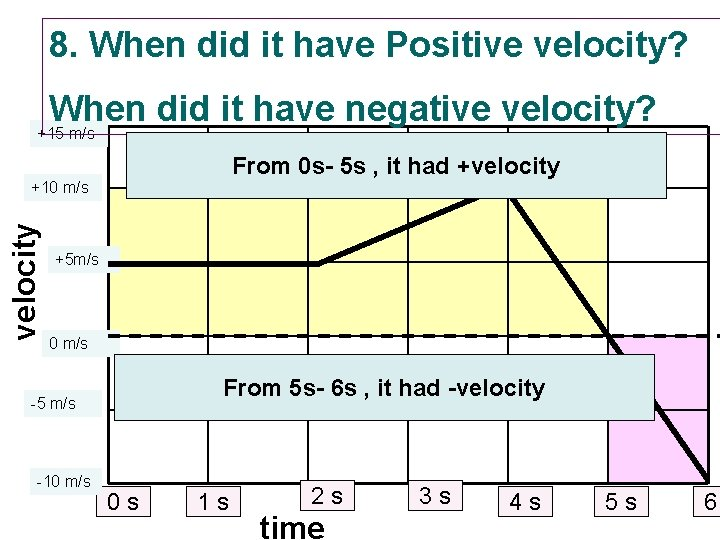8. When did it have Positive velocity? When did it have negative velocity? +15 m/s From 0 s- 5 s , it had +velocity +10 m/s +5 m/s 0 m/s From 5 s- 6 s , it had -velocity -5 m/s -10 m/s 0 s 1 s 2 s time 3 s 4 s 5 s 6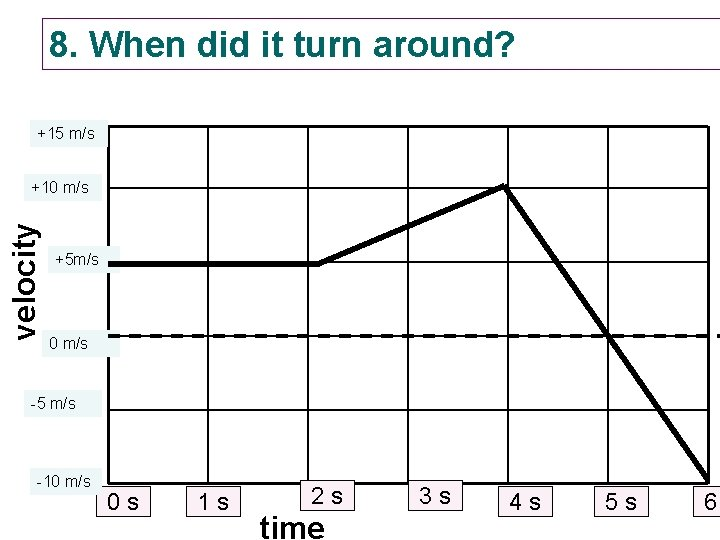8. When did it turn around? +15 m/s velocity +10 m/s +5 m/s 0 m/s -5 m/s -10 m/s 0 s 1 s 2 s time 3 s 4 s 5 s 6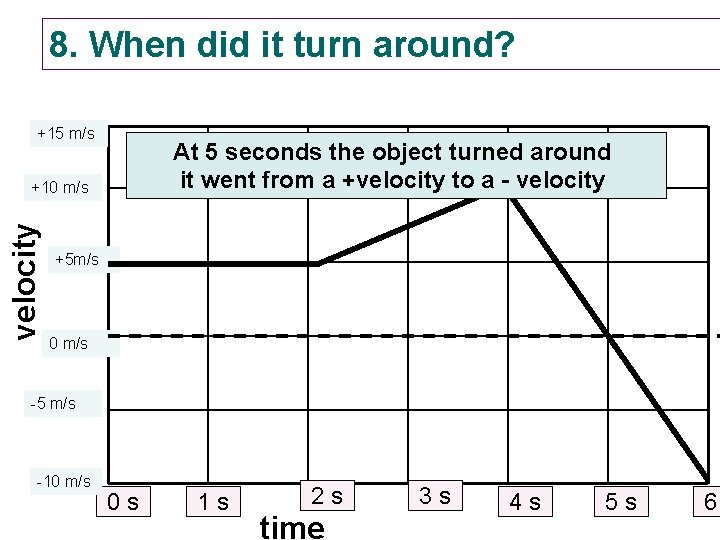8. When did it turn around? +15 m/s At 5 seconds the object turned around it went from a +velocity to a - velocity +10 m/s +5 m/s 0 m/s -5 m/s -10 m/s 0 s 1 s 2 s time 3 s 4 s 5 s 6• Use the next two slides as practice • Answers to follow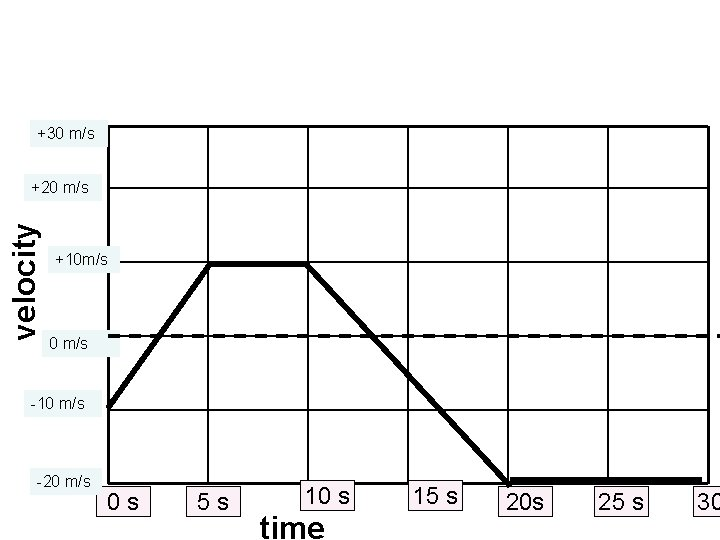+30 m/s velocity +20 m/s +10 m/s 0 m/s -10 m/s -20 m/s 0 s 5 s 10 s time 15 s 20 s 25 s 30Questions 1. How fast is the object moving at 0 s ? How fast is it moving at 2. 5 s ? 5. How fast is the object moving at 20 s? Describe the objects motion from 0 to 2. 5 s How fast is it moving at 30 s ? 2. How fast is the object moving at 2. 5 s? How fast is it moving at 5 s ? Describe the objects motion from 2. 5 s to 5 s Describe the objects motion from 20 to 30 s 4. When did the object travel at constant velocity? What was the velocity? 3. How fast is the object moving at 5 s? How fast is it moving at 10 s ? Describe the objects motion from 5 to 10 s 6. When did the object speed up? How much did it speed up in the time interval? 7. When did the object slow down? 4. How fast is the object moving at 10 s? How fast is it moving at @13 s When did it stop? Describe the objects motion from 10 to 13 s 8. When did it have Positive velocity? 5. How fast is the object moving at 13 s? When did it have negative velocity? How fast is it moving at 20 s Describe the objects motion from 13 to 20 s When did it turn around?Questions 1. How fast is the object moving at 0 s ? -10 m/s 5. How fast is the object moving at 20 s? – 20 m/s How fast is it moving at 2. 5 s ? 0 m/s Describe the objects motion from 0 to 2. 5 s Slowing down to a stop, in a negative direction How fast is it moving at 30 s ? -20 m/s Describe the objects motion from 20 to 30 s Maintaining a constant velocity, in a negative direction 2. How fast is the object moving at 2. 5 s? 0 m/s How fast is it moving at 5 s ? 10 m/s Describe the objects motion from 2. 5 s to 5 s Speeding up, from rest, in a positive direction 3. How fast is the object moving at 5 s? +10 m/s How fast is it moving at 10 s ? +10 m/s Describe the objects motion from 5 to 10 s Traveling in a positive direction at a constant velocity of 10 m/s 6. When did the object travel at constant velocity? What was the velocity? ( 5 to 10 s) at +10 m/s ( 20 to 30 m/s) at -20 m/s 7. When did the object speed up? (2. 5 to 5 s) and (13 to 20 s) How much did it speed up in the time interval? 10 m/s and 20 respectfully 8. When did the object slow down? ( 0 to 2. 5 s) (10 to 13 s) 4. How fast is the object moving at 10 s? +10 m/s How fast is it moving at @13 s 0 m/s When did it stop? At 2. 5 and at 13 s Describe the objects motion from 10 to 13 s Slowing down to a stop, while traveling in a positive direction 9. When did it have Positive velocity? (2. 5 to 13 s) 5. How fast is the object moving at 13 s? 0 m/s When did it have negative velocity? (0 to 2. 5) and (13 to 30 s) How fast is it moving at 20 s? -20 m/s Describe the objects motion from 13 to 20 s Speeding up, in a negative direction When did it turn around? at 2. 5 s and again at 13 sThis powerpoint was kindly donated to www. worldofteaching. com http: //www. worldofteaching. com Is home to well over a thousand powerpoints submitted by teachers. This a free site. Please visit and I hope it will help in your teaching